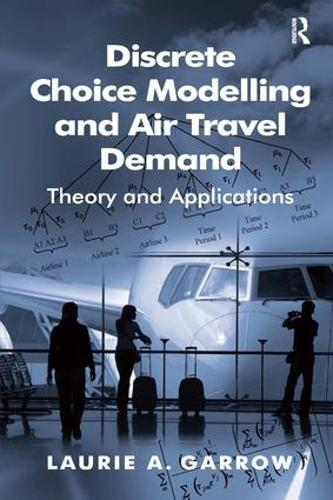•# Discrete Choice Modelling and Air Travel Demand: Theory and Applications (Hardback)

(author)
£120.00
Hardback 306 Pages / Published: 28/06/2010
• We can order this

Usually dispatched within 3 weeks

In recent years, airline practitioners and academics have started to explore new ways to model airline passenger demand using discrete choice methods. This book provides an introduction to discrete choice models and uses extensive examples to illustrate how these models have been used in the airline industry. These examples span network planning, revenue management, and pricing applications. Numerous examples of fundamental logit modeling concepts are covered in the text, including probability calculations, value of time calculations, elasticity calculations, nested and non-nested likelihood ratio tests, etc. The core chapters of the book are written at a level appropriate for airline practitioners and graduate students with operations research or travel demand modeling backgrounds. Given the majority of discrete choice modeling advancements in transportation evolved from urban travel demand studies, the introduction first orients readers from different backgrounds by highlighting major distinctions between aviation and urban travel demand studies. This is followed by an in-depth treatment of two of the most common discrete choice models, namely the multinomial and nested logit models. More advanced discrete choice models are covered, including mixed logit models and generalized extreme value models that belong to the generalized nested logit class and/or the network generalized extreme value class. An emphasis is placed on highlighting open research questions associated with these models that will be of particular interest to operations research students. Practical modeling issues related to data and estimation software are also addressed, and an extensive modeling exercise focused on the interpretation and application of statistical tests used to guide the selection of a preferred model specification is included; the modeling exercise uses itinerary choice data from a major airline. The text concludes with a discussion of on-going customer modeling research in aviation. Discrete Choice Modelling and Air Travel Demand is enriched by a comprehensive set of technical appendices that will be of particular interest to advanced students of discrete choice modeling theory. The appendices also include detailed proofs of the multinomial and nested logit models and derivations of measures used to represent competition among alternatives, namely correlation, direct-elasticities, and cross-elasticities.

Publisher: Taylor & Francis Ltd
ISBN: 9780754670513
Number of pages: 306
Weight: 703 g
Dimensions: 234 x 156 mm